Next: 6. Little Theories Up: 1. Introductory Material Previous: 4. On-Line Help

Subsections

# 5.1 Introduction

This chapter describes about a dozen self-guided, content-based exercises to illustrate the use of IMPS. Each exercise uses a fairly self-contained piece of mathematics, logic, or computer science to show how to organize and develop a theory in IMPS, and how to carry out the proofs needed for the process. We will try to describe in each case the main content of the exercise, as well as the specific techniques that it is intended to illustrate.

We assume in this chapter that you are using the Lucid 19 menu system.

## 5.1.1 How to Use an Exercise

In order to start an exercise, press down any mouse button while pointing to the IMPS-Help menubar item. Release the button while pointing to the entry Exercises. IMPS will present a pop-up menu listing the file names of the available exercises. Clicking on a file name will cause the following actions:

• The system will create a new copy of the file under your own directory, in the subdirectory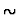/imps/theories. Since this is a new file, you can safely edit it or delete it. If a file of the expected name already exists, IMPS will prompt whether to overwrite the file, thus destroying the previous copy.

• IMPS will create a new Emacs buffer displaying the copy. You will use this buffer to view and edit the file.

• IMPS will search for the first item in the file meriting your attention. Each such item will be marked by the string (!). These items may be candidate theorems to prove, theory declarations or definitions to read, or macetes or scripts to create.

• Anything above the first (!) will be sent as input to the underlying T process. Thus, IMPS will define any notions mentioned at the top of the file.

At any point in the exercise, to proceed to the next item of interest, select--from the IMPS-Help menubar item--the Current Exercise: Next Entry request.
• IMPS will search for the next item in the file marked by the string (!).
• Anything beyond the first (!), up to the current occurrence, will be sent as input to the underlying T process. Thus, IMPS will install the definitions, theorems, and so forth described in that portion of the file.
If you want to restart an exercise in a clean way, click on Exit IMPS under General, and then on Run IMPS. The system will prompt you to confirm the desired amount of virtual memory; the default is plenty for current purposes. Having started a fresh invocation of IMPS, put your cursor in the buffer that you want to work on. Click on the item Restart Exercise in Current Buffer under IMPS-Help.

## 5.1.2 Paths Through the Exercises

The exercises divide roughly into four tiers of difficulty, which are summarized in Table 5.1. We recommend that you do one or more exercises in a tier before moving on to those in the next tier.

Table 5.1: Exercise Files by Tier''
 Tier Exercise file name Section 1. primes-exercise.t 5.2.1 calculus-exercise.t 5.2.2 2. indicators-exercise.t 5.3.1 monoid-exercise.t 5.2.3 3. compiler.t 5.4.1 limits.t 5.2.4 contraction.t 5.2.5 groups-exercise.t 5.2.6 4. flatten.t 5.4.2 temporal.t 5.3.2

# 5.2 Mathematical Exercises

One of the main goals of the IMPS system is to support the rigorous development of mathematics. We hope that it will come to serve as a mathematical data base, storing mathematical concepts, theories, theorems, and proof techniques in a form that makes them available when they are relevant to new work. We believe that as a consequence IMPS will develop into a system that can serve students as a general mathematics laboratory. In addition, we hope it will eventually serve as a useful adjunct in mathematics research.

## 5.2.1 Primes

#### 5.2.1.0.1 Contents.

This exercise introduces two main definitions into the theory of real arithmetic, namely divisibility and primality. It then includes a few lemmas, which lead to a computational'' macete 5.1 to determine whether a positive integer is prime. After introducing the notion of twin primes, the exercise develops two proof scripts that can be used to find a twin prime pair in a given interval, if one exists.

A more extensive treatment of primes, including the material of this exercise, is included in the IMPS theory library in the file

$IMPS/theories/reals/primes.t. That file also contains statements and proofs of the following: • the infinity of primes; • the main properties of gcd; • the existence and uniqueness of prime factorizations (the Fundamental Theorem of Arithmetic). #### 5.2.1.0.2 Purpose. This exercise illustrates basic definitions, and it illustrates how to carry out several simple proofs. Its main interest however is to illustrate the mix of computation and proof in IMPS. This balance is reflected in the macete mechanism, and in the script that searches for a pair of twin primes. ## 5.2.2 A Very Little Theory of Differentiation #### 5.2.2.0.1 Contents. This file develops a little axiomatic theory of the derivative operator on real functions. Its axioms include the rules for: • sum; • product; • constant functions; • the identity function; as well as the principle that the derivative of f is defined at a value xonly if f is. Naturally, these properties hold true of the derivative as explicitly defined in the usual way. The exercise then develops a succession of consequences of these properties, such as the power rule shown in Figure 5.1.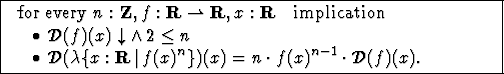The properties and their consequences are then grouped together into a macete that allows expressions involving derivatives to be evaluated. #### 5.2.2.0.2 Purpose. This exercise is intended to illustrate, in addition to inductive reasoning, the manipulation of higher-order objects (functions represented using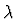), and the power of macetes to encode symbolic computations. ## 5.2.3 Monoids #### 5.2.3.0.1 Contents. This exercises introduces a theory of monoids, a monoid being a domain U equipped with an associative operator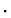and an identity element e. A recursive definition introduces an iterated monoid product operator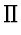, such that, when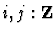and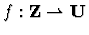, then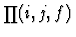satisfies: •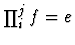when j<i; •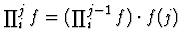when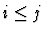. By adding an inverse operator and axioms on it, the monoid theory is extended to a theory of groups (defined independently of the theory in Exercise 5.2.6). The file then defines (slightly incongruously) minus to mean: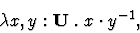and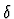to mean: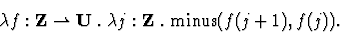That is, if f is a function of sort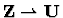, then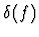is another function of the same sort, and its value for an integer j is the difference (in the sense of minus'') between f(j+1) and f(j). We can then prove the telescoping product formula shown in Figure 5.2.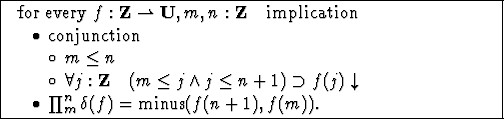This in turn can serve as the basis of an alternative proof for formulas such as the ones for the sum of the first n natural numbers or of their squares. To do so, we introduce an interpretation mapping U to R and the monoid operatorto real addition. As a consequence, the operatorwithin the monoid is carried to the (real-valued) finite summation operator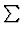. #### 5.2.3.0.2 Purpose. This exercise illustrates how to develop little theories and how to apply them via interpretation. It also illustrates a simple recursive definition. ## 5.2.4 Some Theorems on Limits #### 5.2.4.0.1 Contents. This exercise defines the limit of a sequence of real numbers. A sequence of reals is represented in IMPS as a partial function mapping integers to real numbers. The limit is defined using the iota constructor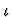, where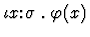has as its value the unique value of x such that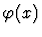holds true. The iota-expression is undefined if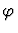is satisfied by more than one value or if it is not satisfied for any value. In this case, the instance ofis the familiar property that, for every positive real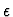, there is an integer n, such that for indices p greater than n,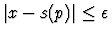. Theorems include a variant of the definition in which iota does not occur, as well as the familiar condition for the existence of the limit. The homogeneity of limit is also proved. #### 5.2.4.0.2 Purpose. The main purpose of this exercise is to illustrate how to reason with iota. In particular, it illustrates the importance of deriving an iota-free'' characterization for a notion defined in terms of iota. ## 5.2.5 Banach's Fixed Point Theorem #### 5.2.5.0.1 Contents. This extensive file, contraction.t, contains a complete proof of Banach's contractive mapping fixed point theorem. An alternative proof using a little more general machinery is contained in the theory library in file$IMPS/theories/metric-spaces/fixed-point-theorem.t

The theorem states that in a complete metric space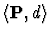, any contractive function has a unique fixed point. A function f is contractive if, there exists a real value k<1 such that for all points xand y in P,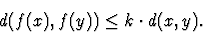The heart of the proof is to show that under this condition the sequence sof iterates of f starting from an arbitrary point x,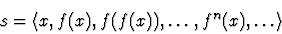will converge. It is then easy to show that the limit of s is a fixed point. Finally, if any two distinct points x and y were both fixed, then we would have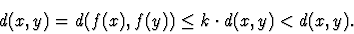This theorem serves as a very good illustration of IMPS's little theories'' approach. It combines:

• Concrete theorems about the reals, such as the geometric series formula, which are needed to bound the differences between points in s;
• A few abstract facts about the sequence of iterates of a function, which are perfectly generic and independent of the fact that we will eventually apply them to functions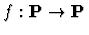;
• A third group of theorems proved in the theory of a metric space, applying the previous theorems.

#### 5.2.5.0.2 Purpose.

The exercise has three purposes:
• To illustrate the little theories approach and how natural it is in mathematics;
• To illustrate inductive proof (in combination with some other techniques) in real arithmetic and also in the generic theory covering iteration;
• To provide a richer, more extended example of how to develop a substantial proof using IMPS.

## 5.2.6 Basics of Group Theory

#### 5.2.6.0.1 Contents.

This file develops the very rudiments of group theory, only up to a definition of subgroup and a proof that subgroups are groups. Most of the content is devoted to building up computational lemmas. This exercise covers only a very small part of the portion of group theory that is developed in the IMPS theory library, in the files contained in the directory:
$IMPS/theories/groups/ #### 5.2.6.0.2 Purpose. The purpose of this exercise is to illustrate how to handcraft the simplification mechanism by proving rewrite rules. In addition, a compound macete is introduced to carry out computations not suited to the simplifier. The file also illustrates the use of a symmetry translation mapping the theory to itself. This translation maps the multiplication operatorto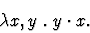Thus, for instance, right cancellation follows by symmetry from left cancellation. # 5.3 Logical Exercises ## 5.3.1 Indicators: A Representation of Sets #### 5.3.1.0.1 Contents. An indicator is a function used to represent a set. It has a sort of the form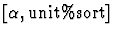, where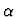is an arbitrary sort. (In the string syntax, the sortis printed as sets[].) unit%sort is a type in the-kernel-theory that contains exactly one element named an%individual. The type is of kind, so indicators may be partial functions. Since the-kernel-theory is a component theory of every IMPS theory, unit%sort is available in every theory. Indicators are convenient for representing sets. The basic idea is that a set s containing objects of sortcan be represented by an indicator of sort, namely the one whose domain is s itself. Simplifying expressions involving indicators is to a large extent a matter of checking definedness--for which the simplifier is equipped with special-purpose algorithms. The theory indicators consists of just a single base sort U, for the universe'' of some unspecified domain. Since the theory indicators contains no constants nor axioms, theory interpretations of indicators are trivial to construct, and thus theorems of indicators are easy to use as transportable macetes. The logical operators in IMPS are fixed, but quasi-constructors can in some respects effectively serve as additional logical operators. Quasi-constructors are desirable for several reasons: • Once a quasi-constructor is defined, it is available in every theory whose language contains the quasi-constructor's home language. That is, a quasi-constructor is a kind of global constant that can be freely used across a large class of theories. (A constructor, which we also call a logical constant, is available in every theory.) • Quasi-constructors are polymorphic in the sense that they can be applied to expressions of several different types. (Several of the constructors, such as = and if, are also polymorphic in this sense.) • Quasi-constructors are preserved under translation. Hence, to reason about expressions involving a quasi-constructor, we may prove laws involving the quasi-constructor in a single generic theory. The translation mechanism can then be used to apply the theorems to expressions in any theory that involve the same quasi-constructor. • Quasi-constructors can be used to represent operators in nonclassical logics, such as modal or temporal logics (see Section 5.3.2), and operators on generic objects such as sets (as represented by indicators) and sequences. Quasi-constructors are implemented as macro/abbreviations.'' The IMPS reader treats them as macros which cause the creation of a particular syntactic pattern in the expression, while the IMPS printer is responsible for printing them in abbreviated form (wherever those patterns may have arisen). The exercise derives a few facts about sets, their unions, and the inclusion relation. It applies them to intervals of integers. #### 5.3.1.0.2 Purpose. The purpose of this exercise is to provide experience reasoning with quasi-constructors. ## 5.3.2 Temporal Logic #### 5.3.2.0.1 Contents. Temporal logic is a kind of modal logic for reasoning about dynamic phenomenon. In recent years temporal logic has won favor as a logic for reasoning about programs, particularly concurrent programs. The following is an exercise to develop a version of propositional temporal logic ( PTL) in IMPS. In this exercise we view time as discrete and linear; that is, we identify time with the integers. The goal of the exercise is to build machinery for reasoning about time predicates,'' which are simply expressions of sort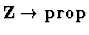. In traditional PTL, the time argument is suppressed and objects which are syntactically formulas are manipulated instead of syntactic predicates. In IMPS we will deal directly with the predicates. This is a very open-ended exercise, which should be done after the user is fairly familiar with IMPS and its modus operandi. # 5.4 Exercises related to Computer Science IMPS also provides some facilities for reasoning about computing applications, although these are still less extensive than those for mathematics. The exercises illustrate a facility for defining recursive data types, as well as an application of a theory of state machines. ## 5.4.1 The World's Smallest Compiler #### 5.4.1.0.1 Contents. This exercise introduces two syntaxes as abstract data types. Each syntax is given a semantics as a simple programming language. A function defined by primitive recursion on one language (the source language'') maps its expressions to values in the other (the target language''). This function is the compiler. We prove that the semantics of its output code matches the semantics of its input. #### 5.4.1.0.2 Purpose. The primary purpose of this exercise is to illustrate the BNF mechanism for introducing a recursive abstract data type. Connected with a BNF is a schema for recursion on the data type. This is a form of primitive recursion, as distinguished from IMPS's more general facility for monotone inductive definitions. The primitive recursion schema is preferable when it applies because it automatically creates rewrite rules for the separate syntactic cases. A secondary purpose is to illustrate in a very simple case an approach to compiler verification. ## 5.4.2 A Linearization Algorithm for a Compiler #### 5.4.2.0.1 Contents. A linearizer is a compiler component responsible for transforming a tree-like intermediate code into a linear structure. Its purpose is typically to transform (potentially nested) conditional statements into the representation using branch and conditional branch instructions that take numerical arguments. The exercise is contained in the file flatten.t. It also depends on the BNF and primitive recursion mechanisms. However, the proofs have more interesting induction hypotheses than in the world's smallest compiler, and the development calls for an auxiliary notion of regular'' instruction sequences. #### 5.4.2.0.2 Purpose. This exercise can be used as a more realistic and open-ended example of a computer science verification. ## 5.4.3 The Bell-LaPadula Exercise #### 5.4.3.0.1 Contents. This exercise consists of two files, namely: •$IMPS/theories/exercises/fun-thm-security.t
The first file proves the Bell-LaPadula Fundamental Theorem of Security'' in the context of the theory of an arbitrary deterministic state machine with a start state, augmented by an unspecified notion of secure state.'' (A similar theorem also holds for nondeterministic state machines.) The proof is by induction on the accessible states of the machine.

The second file instantiates this theory in the case of a simple access control device (reference monitor). The states are functions, which, given a subject and an object returns a pair; one component of the pair represents whether the subject has current read access to the object, while the other represents current write access. The operations of the machine correspond to four requests by a subject concerning an object; the request may be to add or delete and access, which may in turn be either read or write access. The subjects and objects have security levels, and the requests are adjudicated based on their levels.

The theory exploits the fact that read and write are duals, when the sense of the partial ordering on levels is inverted. This duality is expressed by means of a theory interpretation mapping the theory to itself. It is used to manufacture definitions; for instance, the *-property is defined to be the dual of the simple security property. It is also used to create new theorems. For instance, the theorem that the *-property is preserved under get-write is the dual of the theorem that the simple security property is preserved under get-read; hence, it suffices to prove the latter, and the former follows automatically.

#### 5.4.3.0.2 Purpose.

This exercise has three main purposes:
• To illustrate how to extend and to apply the state machine theory, and how to prove theorems using state machine induction;
• To illustrate the use of an interpretation from a theory to itself to encode a symmetry'' or duality;''
• To provide a model for a familiar kind of security verification.Next: 6. Little Theories Up: 1. Introductory Material Previous: 4. On-Line Help